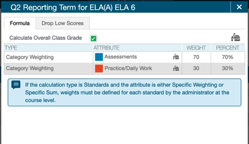1. Home
2. Knowledge Base
3. Educational Data and Technology
4. Assignment Weights in PowerTeacher Pro

## Assignment Weights in PowerTeacher Pro

PowerTeacher Pro enables teachers the ability to assign “extra weight” to an assignment.  This is typically used when you want to increase or lessen the weight of a given assignment within a grading category.

For example, if you’d like a specific assignment to be worth double points in the category total, you would assign a weight of 2.0.  As shown in Figure 1 below, the Chapter 14 Questions assignment has been set a point score value of 4 with a weight of 0.3 which results in this assignment being worth only 1.2 points in the total Practice/Daily Work category average.Figure 1: Assignment with Extra Weight Applied – 0.3

This feature should not be used in place of final grade calculation formulas.  For example, if Assessments are worth 70% and Practice/Daily Work worth 30% of a grading term’s final grade, you should not use assignments weights to achieve this goal.

Final grade calculation weights are achieved via grade calculation formulas managed at the school\district level.  These formulas may be reviewed within PowerTeacher Pro by navigating to Settings | Traditional Grade Calculations and then selecting the term you wish to view.  Figure 2 below shows the final grade calculation formula for the Q2 reporting term, with Assessments worth 70% of the final grade and Practice/Daily Work worth 30% of the final grade.Figure 2: Grade Calculation Weights for Q2 Reporting Term

In summary, assignments should not have a weight assignment to them unless you want to adjust the weight of a specific assignment within a grading category as a special case.